# Calculus 2 : Harmonic Series

## Example Questions

### Example Question #1 : Harmonic Series

The Harmonic series is a special case of a-series, withequal to what?

Possible Answers: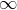Correct answer:Explanation:-series is a series of the form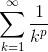, and the Harmonic Series is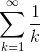. Hence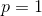.

### Example Question #2 : P Series

Which of the following tests will help determine whether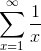is convergent or divergent, and why?

Possible Answers:Nth Term Test: The series diverge because the limit asgoes to infinity is zero.

P-Series Test: The summation converges since.

Integral Test: The improper integral determines that the harmonic series diverge.

Root Test: Since the limit asapproaches to infinity is zero, the series is convergent.Divergence Test: Since limit of the series approaches zero, the series must converge.

Correct answer:

Integral Test: The improper integral determines that the harmonic series diverge.

Explanation:

The seriesis a harmonic series.

The Nth term test and the Divergent test may not be used to determine whether this series converges, since this is a special case.  The root test also does not apply in this scenario.

According the the P-series Test,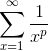must converge only if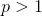.  Therefore this could be a valid test, but a wrong definition as the answer choice since the series diverge for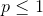.

This leaves us with the Integral Test.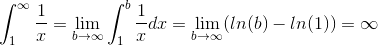Since the improper integral diverges, so does the series.

### Example Question #1 : Alternating Series With Error Bound

Determine whether the following series converges or diverges: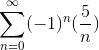Possible Answers:

The series conditionally converges

The series diverges

The series (absolutely) converges

The series may (absolutely) converge, diverge, or conditionally converge

Correct answer:

The series (absolutely) converges

Explanation:

Given just the harmonic series, we would state that the series diverges. However, we are given the alternating harmonic series. To determine whether this series will converge or diverge, we must use the Alternating Series test.

The test states that for a given series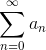where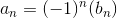or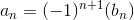where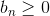for all n, if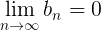and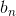is a decreasing sequence, thenis convergent.

First, we must evaluate the limit ofas n approaches infinity: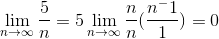The limit equals zero because the numerator of the fraction equals zero as n approaches infinity.

Next, we must determine ifis a decreasing sequence.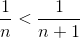, thus the sequence is decreasing.

Because both parts of the test passed, the series is (absolutely) convergent.

### Example Question #4 : Harmonic Series

Consider the alternating series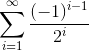.

Which of the following tests for convergence is NOT conclusive?

Possible Answers:

The alternating series test

The limit test for divergence

The root test

The ratio test

Correct answer:

The limit test for divergence

Explanation:

Let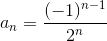be the nth summand in the series. The limit test for divergence states that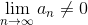implies that the series diverges.

However,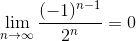,

so the test is inconclusive.

### Example Question #5 : Harmonic Series

Does the following series converge?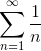Possible Answers:

No

Cannot be determined

Yes

Correct answer:

No

Explanation:

No the series does not converge. The given problem is the harmonic series, which diverges to infinity.

### Example Question #6 : Harmonic Series

Does the following series converge?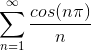Possible Answers:

No

Cannot be determined

Yes

Correct answer:

Yes

Explanation:

The series converges. The given problem is the alternating harmonic series, which converges by the alternating series test.

### Example Question #7 : Harmonic Series

Which of the following tests can be used to (successfully) test for the convergence/divergence of the harmonic series?

Possible Answers:

The Limit Test for Divergence

The Ratio Test

None of the given tests can be used.

The Root Test

The Integral Test

Correct answer:

The Integral Test

Explanation:

Only the Integral Test will work on the Harmonic Series,.

To use the Integral Test, we evaluate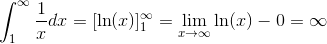, which shows that the series diverges.

Since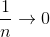, the Limit Test for Divergence fails.

The Ratio Test and the Root Test will always yield the same conclusion, so if one test fails, the both fail and vise versa.

For the Ratio Test,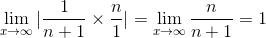. Since the result of the limit is, both tests fail.

### Example Question #8 : Harmonic Series

Let's say you are given harmonic series in the following form: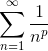;

You are then asked to determine if the series converges, or diverges. For what values of p would this series be convergent? Assume p>0.

Possible Answers: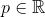Correct answer:Explanation:

The given series is called generalized harmonic series.

The series converges, if, and diverges, if.

### All Calculus 2 Resources